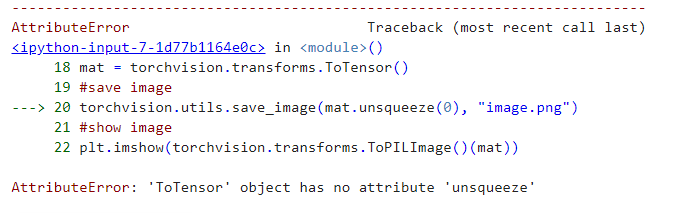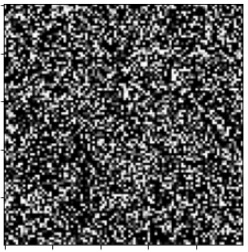# Problem CNN picture

how to display a picture with deep learning

``````print('Validation accuracy test: {:.4f}%'.format(float(accuracy_score(val_y, predictions)) * 100))
conf_matrix  = confusion_matrix(val_y, predictions)
print(conf_matrix)
plt.imshow(torchvision.utils.make_grid(predictions))
``````

Error :

``````TypeError                                 Traceback (most recent call last)
<ipython-input-8-767abd2c3c6d> in <module>()
402
403 print(conf_matrix)
--> 404 plt.imshow(torchvision.utils.make_grid(predictions))

1 frames
44     if not (torch.is_tensor(tensor) or
45             (isinstance(tensor, list) and all(torch.is_tensor(t) for t in tensor))):
---> 46         raise TypeError(f'tensor or list of tensors expected, got {type(tensor)}')
47
48     if "range" in kwargs.keys():

TypeError: tensor or list of tensors expected, got <class 'numpy.ndarray'>
``````

Help me plzzz

`make_grid` expects a 4D tensor or a list of of images as described in the docs:

tensor (Tensor or list) – 4D mini-batch Tensor of shape (B x C x H x W) or a list of images all of the same size.

while you are trying to pass a numpy array to it.

But when I tested this :

``````conf_matrix  = confusion_matrix(val_y, predictions)

print(conf_matrix)

plt.imshow(torchvision.utils.make_grid((predictions, nrow: int = 8, padding: int = 2, normalize: bool = False, value_range: Optional[Tuple[int, int]] = None, scale_each: bool = False, pad_value: int = 0, **kwargs))
``````

Error :

File “”, line 403
plt.imshow(torchvision.utils.make_grid((predictions, nrow: int = 8, padding: int = 2, normalize: bool = False, value_range: Optional[Tuple[int, int]] = None, scale_each: bool = False, pad_value: int = 0, **kwargs))
^
SyntaxError: invalid syntax

It seems you might have just copied the arguments from the docs without defining them.
This would work:

``````predictions = torch.randn(4, 3, 224, 224)
plt.imshow(torchvision.utils.make_grid(predictions).permute(1, 2, 0))
``````

Thank you @ptrblck but how to save the image in extension .mat? plzz

`scipy.io.savemat` should work.

Plzz @ptrblck when I affiched the image with this code , always , it displays under this form

``````mat = pjoin('./sample_data/', 'img.mat')
mat = torch.randn(1, 1, 100, 100)
plt.imshow(torchvision.utils.make_grid(mat).permute(1, 2, 0))
``````

yet it is not this image

help me plzz @ptrblck

Hey @randino ,
You are using `mat` to load your image and again assigning `mat` to a tensor with random value. So, no matter how many times you change the image path, you will get a random image. Let me explain.
This two lines will read the `mat` file.

``````mat = pjoin('./sample_data/', 'img.mat')
``````

So, `mat` is now a numpy array image. But, this line will assign a tensor with random values, overwriting previous `mat` value.

``````mat = torch.randn(1, 1, 100, 100) # you are assigning a random tensor to 'mat' variable
``````

So, when you try to show or save the image, you get a random image.

``````plt.imshow(torchvision.utils.make_grid(mat).permute(1, 2, 0) # this mat is a random tensor
``````

You can try the following.

``````mat = pjoin('./sample_data/', 'img.mat')
## extract image from mat
## .....
## .....
mat = torchvision.transforms.ToTensor()(mat)
#save image
torchvision.utils.save_image(mat, "image.png")
#show image
plt.imshow(torchvision.transforms.ToPILImage()(mat))
plt.show()
``````

but this errorHi @randino ,

in this line the problem

``````mat = torchvision.transforms.ToTensor()(mat)
``````

Hi @randino,
This is expected as `mat` files are `dict` files with `keys` being the `MATLAB` variables and `values` being the objects assigned to those variables.

But when I saved in my computer size (256,256) and whenI read with matlab I find the size change (252, 262)!

Please check if you have any resize operation anywhere in code. Also, check your saving code.

No i just did right button save image

Hi @Sayed_Nadim @ptrblck how I save the image in my computer plzz

Hi @randino ,
Please check the following minimal example on how to read, save and load image from `mat` file.

``````import scipy.io as sio
import matplotlib.pyplot as plt
import numpy as np

# taking the cifar-10 mat version for testing
mat = './cifar-10-batches-mat/data_batch_1.mat'
# finding the key values
print(mat.keys())
# output:  dict_keys(['__header__', '__version__', '__globals__', 'data', 'labels', 'batch_label'])

# we will take the "data" key from the dict as it contains images
data = np.asarray(mat.get('data'))
# reshaping the raw data into image format (channel first approach)
data = data.reshape((-1, 3, 32, 32))
data = data.swapaxes(3, 2).swapaxes(1, 3)  # swapping axes for image correction
data_image = data  # taking the first image of the dataset
print(data_image.shape)
# output: (32,32,3)
plt.imsave('./data_image.jpg', np.asarray(data_image))  # saving the image

print(image.shape)
# output: (32,32,3)
``````

Let me know if you face any problems.@Sayed_Nadim @ptrblck it did not work

What seems to be the problem?

@Sayed_Nadim I want to display the results of the classification (prediction of the code) in image, how?

I tested by this code which gave by @ptrblck but still displays this image

``````print('Validation accuracy test: {:.4f}%'.format(float(accuracy_score(val_y, predictions)) * 100))
conf_matrix  = confusion_matrix(val_y, predictions)
print(conf_matrix)
predictions = torch.randn(1, 1, 224, 224)
plt.imshow(torchvision.utils.make_grid(predictions).permute(1, 2, 0))
``````